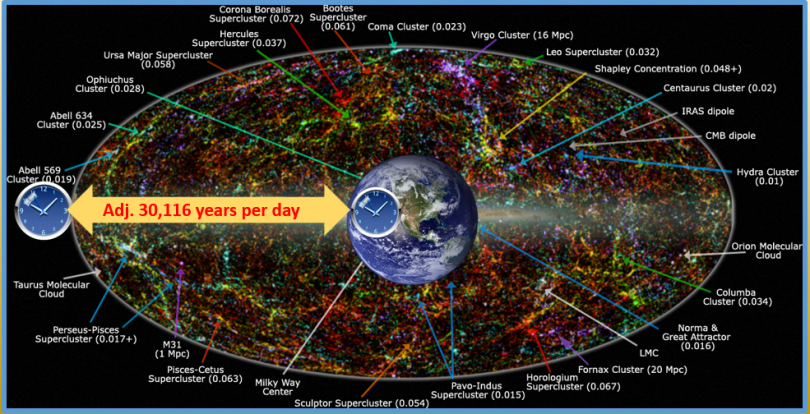# TIME DILATION FINDS TIME MOVES AT DIFFERENT RATESTime dilation finds that time moves at different rates depending on where the observer is located. Such evidence reveals that time and space distance are not dependent on each other. Such a reality gives room for a massively large universe (billions of light years in width) not requiring billions of years to have formed.

(Albert Einstein’s) Time dilation isn’t just theoretical; global-positioning satellites have to take it into effect to provide accurate readings to users here on Earth. And scientists have measured the phenomenon precisely in the field, most famously in 1976 with the Gravity Probe-A experiment. Back then, researchers launched an atomic clock to an altitude of about 6,200 miles (10,000 kilometers), then compared its ticking to that of a similar instrument here on Earth. The results confirmed the predictions of general relativity, to within 0.007 percent.”

https://www.space.com/42641-einstein-gravitational-time-dilation-galileo-probes.html

Britannica: “Time dilation, in the theory of special relativity, the “slowing down” of a clock as determined by an observer who is in relative motion with respect to that clock. In special relativity, an observer in inertial (non-accelerating position) motion has a well-defined means of determining which events occur simultaneously with a given event.  A second inertial observer, who is in relative motion with respect to the first, however, will disagree with the first observer regarding which events are simultaneous with that given event.

Neither observer is wrong in this determination; rather, their disagreement merely reflects the fact that simultaneity is an observer-dependent notion in special relativity.  A notion of simultaneity is required in order to make a comparison of the rates of clocks carried by the two observers. If the first observer’s notion of simultaneity is used, it is found that the second observer’s clock runs slower than the first observer’s… Similarly, using the second observer’s notion of simultaneity, it is found that the first observer’s clock runs slower by the same factor.  Thus, each inertial observer determines that all clocks in motion relative to that observer run slower than that observer’s own clock.”

Derived from teaching by bible teacher Chuck Missler:

Using the example of the adjustments made to the atomic clocks on earth (Greenwich and Boulder, Colorado) each year we can apply the math to distant starlight from the edge of the observable universe.  Using the same math, we apply these same adjustments and find an observer difference of 3.88 hours per day or 5,098,320 seconds per year.  (We keep this in seconds because the speed of light travels in miles per second).  The Speed of light travels at 186,282 miles per second.

Therefore, the observable time adjustment based on Einstein’s time dilation formula as applied from earth to the edge of the observable universe separates an astounding 9.49 X 1011 miles per year!   This is an addition to the rate of time as it actually passes on earth– from our observational point.  There are many problems with this theory as an example, the universe is perhaps expanding at increasing rates.  However, this conjecture (opinion) indicates that the ages affixed to the distant starlight of the universe which naturalists theorize as 13.8 billion years ‘space/time’ actually indicate a much younger universe.

Another way of stating this is the clocks between the edge of the universe and earth (assuming a biblical timeframe of approximately six thousand years) requires a time dilation adjustment of 180 million years!

While it is not surprising that these calculations don’t fall into biblical (or naturalistic) time frames (because the creation event was a miraculous and did not happen by naturalistic events) these adjustments do bring serious questions to the billions of years assumed by evolutionary timelines and indicate the universe may be young enough to meet biblical time frames.

https://www.britannica.com/science/time-dilation

http://cobalt.rocky.edu/~hyla.thompson/bible_notes/01%20Genesis%20Notes.pdf
https://www.scientificamerican.com/article/einsteins-time-dilation-prediction-verified/